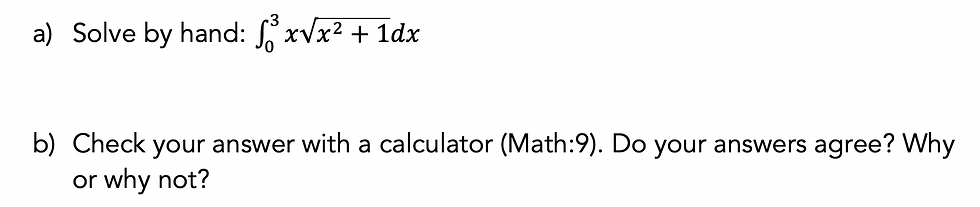top of page
Search

# 8 Strategies to Build Understanding of U-substitution

U-substitution is notoriously tricky for students (maybe because it’s directly linked to another challenging concept, the chain rule!) Oftentimes, it seems like the only option is to accept the initial confusion and blank stares and then hope they’ll catch on after doing enough practice problems. We think there’s a better way.

By carefully crafting experiences that help motivate the need for u-substitution, we’ve seen students gain much better conceptual understanding for when, why, and how to use u-substitution. Most of these strategies are short and are done in a warm-up that takes less than 5 minutes.

The strategies below are meant to be done in order. The first four strategies prime students for u-substitution and are designed to be done before any formal learning on u-substitution occurs (they don’t know that term yet either!) The 5th through 7th strategy are designed for students to experience and formalize their learning on u-substitution for indefinite and definite integrals. The last strategy is used for students to practice u-substitution in a purposeful way.

1. Find derivatives using the chain rule. As a warm-up, have students find y' for each of the functions shown below.Goal: Help students recall how to take derivatives of composite functions.

2. Emphasize the connection between derivatives and antiderivatives with the following warm-up.While these questions may seem trivial, we think they're an important step in connecting the processes of chain rule differentiation and u-substitution. We hope students find these questions easy and a little bit silly!

Goal: Reinforce that finding an antiderivative is simply undoing the process of differentiation. It answers the question: “What function would have this function as its derivative?”

3. Ask students to find an antiderivative of a function that is clearly a result of using the chain rule. Trig functions and exponential functions work well for this because there is not likely to be a simplification of terms. Using the dot notation for multiplication further primes students to see the two links in the chain.Goal: Help students recognize integrand functions that are the direct result of using the chain rule.

4. Error analysis: Give a sample answer where a student's answer is off by a correction factor. Ask students to explain where, if at all, the student made a mistake. Students should be able to show that the derivative of the antiderivative does not match the integrand. They do not yet have the language of a “correction factor”, but they will should still be able to revise the student’s answer based on logic and reasoning.Goal: Reinforce the idea that the derivative of the antiderivative must give back the integrand function. Plant seeds for a “correction factor.”

5. Actually teach u-substitution (but only for indefinite integrals).

Our Experience First, Formalize Later (EFFL) lesson uses a “Which One Doesn’t Belong?” approach to consolidate all the previous exercises and have students experience u-substitution, before formalizing with the steps of a more generalized approach. (Read more about why we think the EFFL model is the right approach to teaching AP Calculus).

6. Go really slow. Break it down. When you first give students practice problems with u-substitution, don’t expect they can do them independently from start to finish. Break down the process and isolate the key skills.

We gives several practice problems where students only have to

1. identify “u”

2. find du/dx and then

3. recognize if an exact match for du/dx is already in the integrand or not

Goal: Recognize integrand functions that are the result of using the chain rule, identify inside and outside functions, and motivate the need for seeing the du/dx term.

7. Extend u-substitution to definite integrals by breaking their tools. This is when we give students a question they are likely to get wrong to expose a problem and motivate the need for a new strategy. Here, we give students the following warm-up, where we ask students to evaluate a definite integral knowing they will likely use “u” terms and “x” terms simultaneously, leading to an incorrect answer.This warm-up problem leads into a discussion about two correct methods for evaluating definite integrals, one that requires adjusting the limits of integration (putting everything into u-terms) and one that doesn’t (substituting back in the expression for “u” so that everything is in terms of x). We explore why both methods give equivalent results and ask students which method they prefer.

Goal: Provide an experience that reveals a common mistake when evaluating definite integrals and learn methods for how to avoid it.

8. Purposeful practice: u-substitution is tricky for students! They need meaningful opportunities to practice. But that doesn’t mean they have to do countless worksheets. Instead, we made this u-sub scavenger hunt to provide students with rich problems in an engaging format.

Goal: You guessed it. Practice.